Courses

# RD Sharma Solutions -Ex-4.4, Operations On Whole Numbers, Class 6, Maths Class 6 Notes | EduRev

## RD Sharma Solutions for Class 6 Mathematics

Created by: Abhishek Kapoor

## Class 6 : RD Sharma Solutions -Ex-4.4, Operations On Whole Numbers, Class 6, Maths Class 6 Notes | EduRev

The document RD Sharma Solutions -Ex-4.4, Operations On Whole Numbers, Class 6, Maths Class 6 Notes | EduRev is a part of the Class 6 Course RD Sharma Solutions for Class 6 Mathematics.
All you need of Class 6 at this link: Class 6

Q1. Does there exist a whole number ‘a’ such that a / a = a ?

Solution: Yes, there exists a whole number ‘a’ such that a/a = a.

The whole number is 1 such that,

1 / 1 = 1

Q2. Find the value of:

Solution: (i) 23457 / 1 = 23457

(ii) 0 / 97 = 0

(iii) 476 + (840 / 84) = 476 + 10 = 486

(iv)964 - (425 / 425) = 964 - 1 = 963

(v) (2758 / 2758) - (2758 + 2758) = 1 - 1 = 0

(vi) 72450 / (583 - 58) = 72450 + 525 = 138

Q3. Which of the following statements are true:

Solution: (i) False

LHS: 10 / (5 x 2)

= 10 / 10

=1

RHS: (10 / 5) x (10 / 2)

= 2 x 5 = 10

(ii) True

LHS: (35 - 14) / 7

= 21 / 7

=3

RHS: 35 / 7 - 14 / 7

= 5 - 2 = 3

(iii) False

LHS: 35 - 14 / 7

= 35 - 2 = 33

RHS: 35 / 7 - 14 / 7

= 5 - 2

= 3

(iv)False

LHS: (20 - 5) / 5

= 15 / 5

=3

RHS: 20 / 5 – 5

= 4 - 5 = -1

(v) False

LHS: 12 x (14 / 7)

= 12 x 2

= 24

RHS: (12 x 14) / (12 x 7)

= 168 / 84

=2

(vi) True

LHS: (20 / 5) / 2

= 4 / 2

=2

RHS : ( 20 / 2 ) / 5

= 10 / 5

= 2

Q4. Divide and check the quotient and remainder:

Solution: (i) 7777 / 58 = 134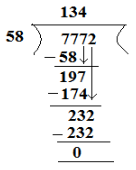Verification: [ Dividend = Divisor x Quotient + Remainder ]

7772 = 58 x 134 + 0

7772 = 7772

LHS = RHS

(ii) 6906 / 35 gives quotient = 197 and remainder = 11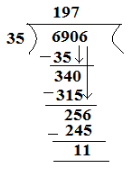Verification: [ Dividend = Divisor x Quotient + Remainder ]

6906 = 35 x 197 + 11

6906 = 6895 + 11

6906 = 6906

LHS = RHS

(iii) 16135 / 875 gives quotient = 18 and remainder = 385.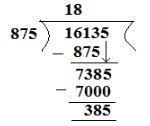Verification: [ Dividend = Divisor x Quotient + Remainder ]

16135 = 875 x 18 + 385

16135 = 15750 + 385

16135 = 16135

LHS = RHS

(iv) 16025/1000 gives quotient and remainder = 25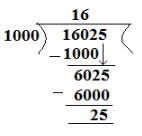Verification: [ Dividend = Divisor x Quotient + Remainder ]

16025 = 1000 x 16 + 25

16025 = 16000 + 25

16025 = 16025

LHS = RHS

Q5. Find a number which when divided by 35 gives the quotient 20 and remainder 18.

Solution: Dividend = Divisor x Quotient + Remainder

Dividend = 35 x 20 + 18

= 700 + 18

= 718

Q6. Find the number which when divided by 58 gives a quotient 40 and remainder 31.

Solution: Dividend = Divisor x Quotient + Remainder

Dividend = 58 x 40 + 31

= 2320 + 31

= 2351

Q7. The product of two numbers is 504347. If one of the numbers is 1591, find the other.

Solution: Product of two numbers = 504347

One of the two numbers = 1591

Let the number be A.

Therefore, A x 1591 = 504347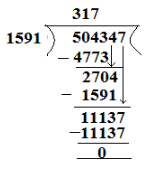A = 5043471591 = 317

Q8. On dividing 59761 by a certain number, the quotient is 189 and the remainder is 37. Find the divisor.

Solution: Dividend = 59761

Quotient = 189

Remainder = 37

Divisor = A

Now, Dividend = Divisor x Quotient + Remainder

59761 =A x 189 + 37

59761 - 37 = A x 189

59724 = A x 18

Therefore, A = 59724189

= 316

Q9. On dividing 55390 by 299, the remainder is 75. Find the quotient.

Solution: Dividend = 55390

Divisor = 299

Remainder = 75

Quotient = A

Dividend = Divisor x Quotient + Remainder

55390 = 299 x A + 75

55390 - 75 = A x 299

55315 = A x 299

Therefore, A = 55315299 = 185

Offer running on EduRev: Apply code STAYHOME200 to get INR 200 off on our premium plan EduRev Infinity!

103 docs

,

,

,

,

,

,

,

,

,

,

,

,

,

,

,

,

,

,

,

,

,

,

,

,

,

,

,

,

,

,

;